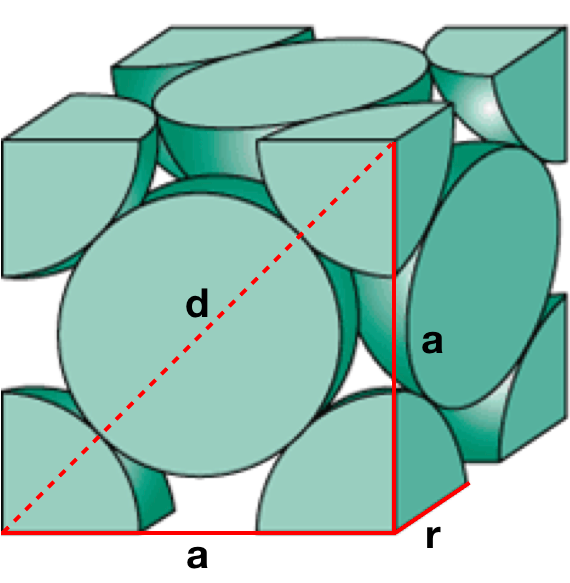# Problem: An element crystallizes in a face-centered cubic lattice. The edge of the unit cell is 4.078×10−8 cm, and the density of the crystal is 19.30 g/cm3. Calculate the atomic weight of the element.

###### FREE Expert Solution

In this problem, we are asked to determine the atomic weight of the element.

Recall that the face-centered cubic lattice contains an atom in each of the face of the cube and an atom in each of the corners

It looks like this:To solve this problem

Step 1. Determine the volume of the cell.

Step 2. Determine the mass of the cell.

Step 3. Determine the number of atoms

Step 4. Calculate the atomic mass of the element.###### Problem Details

An element crystallizes in a face-centered cubic lattice. The edge of the unit cell is 4.078×10−8 cm, and the density of the crystal is 19.30 g/cm3. Calculate the atomic weight of the element.